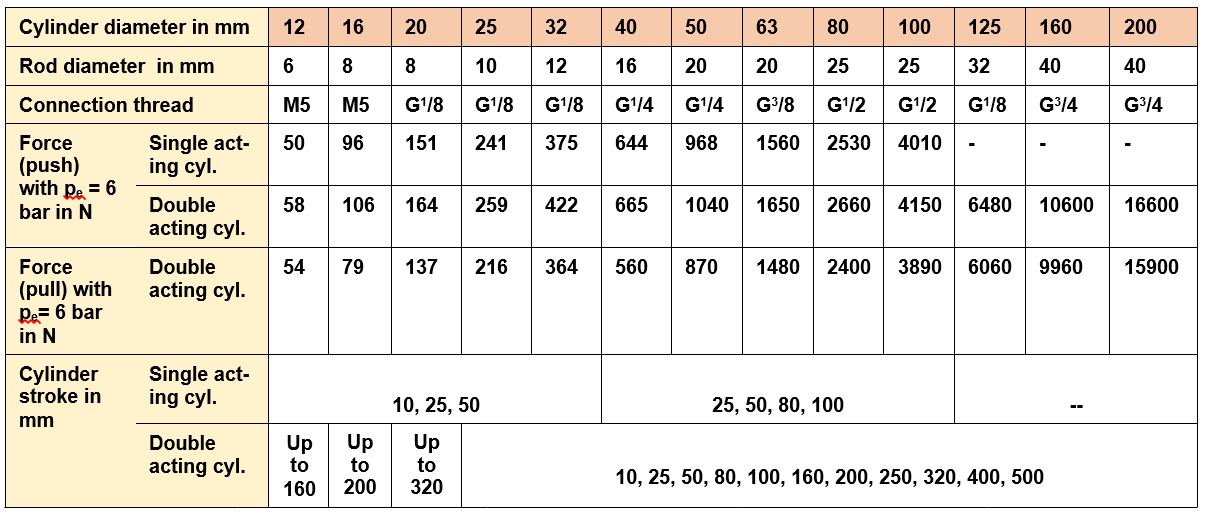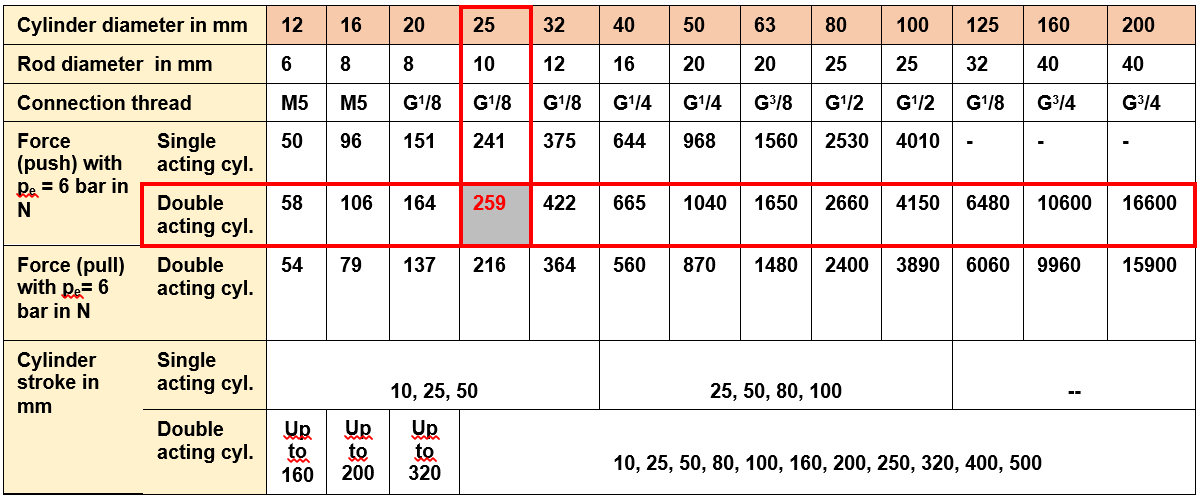# Cylinder force

### Determine cylinder force

The double-acting cylinder has two working ports and can therefore create a force during extending and retracting the piston (hence the naming). The single-acting cylinders, however, can produce a force in one direction only. The retraction is done here by a return spring.

The single-acting cylinder has a significantly worse efficiency simply because the piston force has to work against the return spring. The efficiency is usually indicated on the data sheet of the cylinder. In this script we want, unless otherwise specified, assume an efficiency of 0.95 for double-acting cylinders and 0.8 for single-acting cylinders.

Furthermore single-acting cylinders spoil much faster because air from the environment enters into the cylinder body while retracting. The advantage for the simple-acting cylinders lies in the lower air consumption because the return spring retracts this cylinder automatically.

Determine piston force

The piston force F depends on the working pressure pe, the applied piston area A and the efficiency η:

F = pGauge * A * η

Behind this is the law of Pascal: p = F / A

Units:  1 bar = 105 Pa = 105 N/m2 =  10 N/cm2

Note: Here, in this script we do not consider the rod diameter for reasons of simplicity. This applies to the calculation of the piston force of a double-acting cylinder during retraction. In hydraulics, this would of course be inadmissible.

4A1:   Work order  `Laserstation´Introduction - Determine piston force

For labeling workpieces by means of a laser, these are raised together with the workpiece carrier by a double-acting cylinder:

The mass, which the cylinder has to lift, is about 26 kg. Which diameter must the cylinder piston have at least at an operating pressure of 6 bar? Determine the cylinder diameter by calculation proof it by using this table! The efficiency according to the manufacturer is about 0.94!Table - Piston force

Weight force of the workpiece:  FG = m * g  = 26 kg * 9,81 m/s2 = 255,06 N  Δ  FMin.Cyl.

FZyl. = p * A * ɳ  │  ɳ = 0,94

=>   A = FZyl./(p * ɳ)   = 255,06 N / (60 N/cm2  * 0,94)  =  4,522 cm2  =  452,2 mm2

A = ᴫ d2/4   =>  d = √ (4 A / ᴫ )   =   √( 4 * 452,2 / ᴫ)  mm   = 23,99 mm

Solution via table:Table - piston force solution